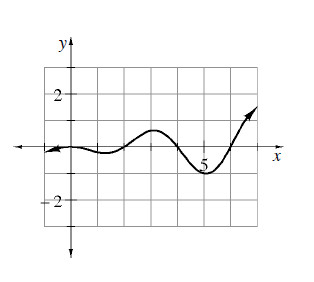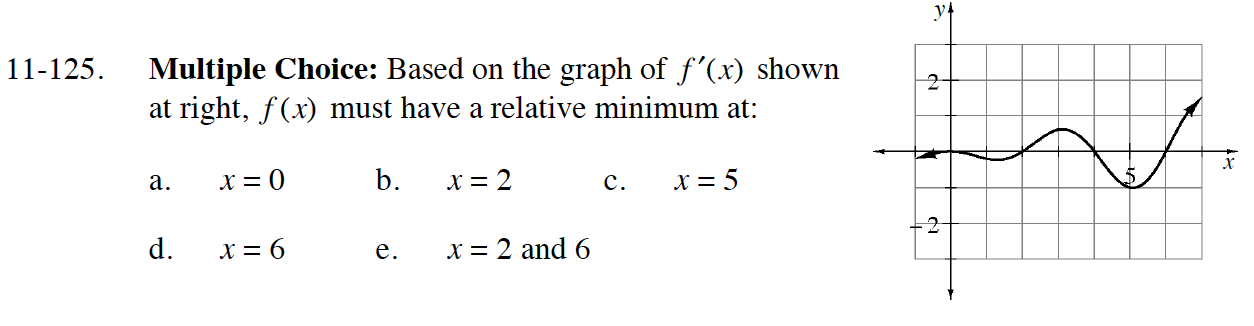### Home > CALC > Chapter 11 > Lesson 11.4.1 > Problem11-125

11-125.
1. Multiple Choice: Based on the graph of f ′(x) shown below, f(x) must have a relative minimum at: Homework Help ✎1. x = 0

2. x = 2

3. x = 5

4. x = 6

5. x = 2 and 6The given graph is a slope graph. A minimum occurs where the slope graph changes from negative to positive.